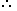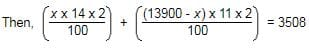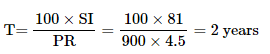# Test: Simple Interest- 1

## 10 Questions MCQ Test CSAT Preparation for UPSC CSE | Test: Simple Interest- 1

Description
Attempt Test: Simple Interest- 1 | 10 questions in 10 minutes | Mock test for Banking Exams preparation | Free important questions MCQ to study CSAT Preparation for UPSC CSE for Banking Exams Exam | Download free PDF with solutions
QUESTION: 1

### Suresh for 2 years invested Rs. 500 in SBI. He also invested Rs. 300 in ICICI for 4 years. At the end he received Rs. 220 from both banks as simple interest. What must have been rate of interest? (assuming the rate of interest for both banks is same)

Solution:

Simple Intrest = SI = PRT/100
Where P = Principal, R = Rate of intrest and T = time period
Total SI = SI from SBI and SI from ICICI
∴ 220 = (500 x R x 2)/100 + (300 x R x 4)/100
⇒ 220 = 10R + 12R
⇒ R = 10% = Rate of intrest

QUESTION: 2

### A sum of money at simple interest amounts to Rs. 815 in 3 years and to Rs. 854 in 4 years. The sum is :

Solution:

S.I. for 1 year = Rs. (854 - 815) = Rs. 39.

S.I. for 3 years = Rs.(39 * 3) = Rs. 117.Principal = Rs. (815 - 117) = Rs. 698.

QUESTION: 3

### A man took loan from a bank at the rate of 8% p.a. simple interest. After 4 years he had to pay Rs. 6200 interest only for the period. The principal amount borrowed by him was:

Solution:

SI = PRT / 100
⇒ (SI x 100) / RT = P
⇒ P = (6200*100) / 32
⇒ P = 19375

QUESTION: 4

Mr. Thomas invested an amount of Rs. 13,900 divided in two different schemes A and B at the simple interest rate of 14% p.a. and 11% p.a. respectively. If the total amount of simple interest earned in 2 years be Rs. 3508, what was the amount invested in Scheme B?

Solution:

Let the sum invested in Scheme A be Rs. x and that in Scheme B be Rs. (13900 - x)⇒ 28x - 22x = 350800 - (13900 * 22)
⇒ 6x = 45000
⇒ x = 7500
So, sum invested in Scheme B = Rs. (13900 - 7500) = Rs. 6400

QUESTION: 5

A sum fetched a total simple interest of Rs. 929.20 at the rate of 8 p.c.p.a. in 5 years. What is the sum?

Solution:

Given, SI = Rs 929.20
P = ?
T = 5 years
R = 8%
P = (100 x SI) / RT
⇒ (100 x 929.20) / (8 x 5)
⇒ Rs. 2323

QUESTION: 6

Arun took a loan of Rs. 1400 with simple interest for as many years as the rate of interest. If he paid Rs.686 as interest at the end of the loan period, what was the rate of interest?

Solution:

Simple Interest (SI) = P N R / 100

P is the Principal loan amount = Rs.1400

N is the number of years of deposit

R is the rate of interest

It is given that the loan period is as many years as the rate of interest.

So, N = R

Interest at the end of the loan period (SI ) = Rs.686

So,

686 = 1400 * R * R /100

R2 = 686*100 /1400

R2 = 49

R = 7%

QUESTION: 7

Q. How much time will it take for an amount of Rs. 900 to yield Rs. 81 as interest at 4.5% per annum of simple interest?

Solution:

Given, P = Rs.900

SI = Rs.81

T = ?

R = 4.5%QUESTION: 8

What will be the ratio of simple interest earned by certain amount at the same rate of interest for 5 years and that for 15 years?

Solution:

Simple Interest = PRT / 100
Here P = Principal, R = Rate of interest and T = time period
Hence, Simple Interest ∝ T
Required Ratio = (Simple Interest for 5 years) / (Simple Interest for 15 years)
⇒ T1/ T2 = 5 / 15
⇒ 1 / 3 = 1 : 3

QUESTION: 9

A sum of money amounts to Rs.9800 after 5 years and Rs.12005 after 8 years at the same rate of simple interest. The rate of interest per annum is

Solution:

We can get SI of 3 years = 12005 - 9800 = 2205

SI for 5 years = (2205/3)*5 = 3675 [so that we can get principal amount after deducting SI]

Principal = 9800 - 3675 = 6125

So Rate = (100*3675)/(6125*5) = 12%

QUESTION: 10

A certain amount earns simple interest of Rs. 1750 after 7 years. Had the interest been 2% more, how much more interest would it have earned?

Solution:

We need to know the S.I, principal and time to find the rate. Since the principal is not given, so data is inadequate.Use Code STAYHOME200 and get INR 200 additional OFF Use Coupon Code